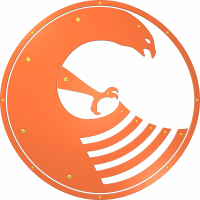# Falcon

Appeared in:
2003
Influenced by:
Typing discipline:
File extensions:
.ftd, .fal, .fam
Versions and implementations (Collapse all | Expand all):
Programming language

Falcon (named after Italian judge Giovanni Falcone) is a multiparadigm programming language which combines as many as six paradigms, including rare tabular and message passing ones.

A prototype of the language called HASTE (from Haste Advanced Simple Text Evaluator) was created by Giancarlo Niccolai in 2002. Later it evolved into a mature language and was renamed to Falcon. In 2008 it was released under open source license, and now the language has gathered a small community of active developers around it, so-called Falcon Committee.

The language is suited both for creating standalone applications and for embedding it in applications in other languages. Program source code is translated into virtual machine instructions which can be regarded both as an interpreter and as an embedding tool.

• procedural. Falcon supports classic functions declarations and calls, as well as a set of structured-style statements.
• functional. Falcon is a second-order language, so it allows passing functions as parameters for other functions. Besides, it has an evaluation engine called Sigma-reductor which allows writing purely functional programs, without any procedural constructs. Functional sequences are represented with arrays, so they can be manipulated at runtime.
• object-oriented, prototype-based, tabular and message passing paradigms are supported in their typical way.Falcon logo

## Examples:

### Hello, World!:

Example for versions Falcon 0.9.6.6
``````printl('Hello, World!')
``````

### Factorial:

Example for versions Falcon 0.9.6.6

This example uses iterative factorial calculation.

``````fact = 1
for i in [0:16]
printl (i, "! = ", fact)
fact *= (i+1)
end
``````

### Factorial:

Example for versions Falcon 0.9.6.6

This example uses recursive factorial calculation.

``````function fact(n)
if n==0: return 1
return n * fact(n-1)
end

for i in [0:16]
printl (i, "! = ", fact(i))
end
``````

### Fibonacci numbers:

Example for versions Falcon 0.9.6.6

This example uses recursive definition of Fibonacci numbers.

``````function fib(n)
if n <= 2 :  return 1
return fib(n-1) + fib(n-2)
end

for i in [1:17]
print (fib(i), ", ")
end
printl ("...")
``````

### CamelCase:

Example for versions Falcon 0.9.6.6

This program processes the input string character by character.

``````text = input().lower()
cc = ""
was_space = true
for i in [ 0 : text.len() ]
if text[i] >= 'a' and text[i] <= 'z'
if was_space
cc += text[i].upper()
else
cc += text[i]
end
was_space = false
else
was_space = true
end
end
printl(cc)
``````

Example for versions Falcon 0.9.6.6
``````a = int(input())
if a == 0
exit()
end
b = int(input())
c = int(input())
d = b ** 2 - 4 * a * c
if d == 0
printl("x = " + (-b / 2.0 / a))
else
if d > 0
printl("x1 = " + ((-b + d**0.5) / 2.0 / a))
printl("x2 = " + ((-b - d**0.5) / 2.0 / a))
else
printl("x1 = (" + (-b / 2.0 / a) + "," + ((-d)**0.5 / 2.0 / a) + ")")
printl("x2 = (" + (-b / 2.0 / a) + "," + (- ((-d)**0.5 / 2.0 / a)) + ")")
end
end
``````